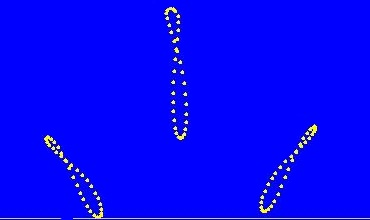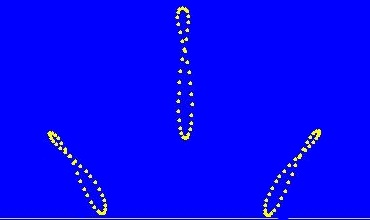# Plotting the Analemma (Shallow Thoughts)

Akkana's Musings on Open Source Computing and Technology, Science, and Nature.

## Plotting the Analemma

My SJAA planet-observing column for January is about the Analemma and the Equation of Time.

The analemma is that funny figure-eight you see on world globes in the middle of the Pacific Ocean. Its shape is the shape traced out by the sun in the sky, if you mark its position at precisely the same time of day over the course of an entire year.

The analemma has two components: the vertical component represents the sun's declination, how far north or south it is in our sky. The horizontal component represents the equation of time.

The equation of time describes how the sun moves relatively faster or slower at different times of year. It, too, has two components: it's the sum of two sine waves, one representing how the earth speeds up and slows down as it moves in its elliptical orbit, the other a function the tilt (or "obliquity") of the earth's axis compared to its orbital plane, the ecliptic.The Wikipedia page for Equation of time includes a link to a lovely piece of R code by Thomas Steiner showing how the two components relate. It's labeled in German, but since the source is included, I was able to add English labels and use it for my article.

But if you look at photos of real analemmas in the sky, they're always tilted. Shouldn't they be vertical? Why are they tilted, and how does the tilt vary with location? To find out, I wanted a program to calculate the analemma.

### Calculating analemmas in PyEphem

The very useful astronomy Python package PyEphem makes it easy to calculate the position of any astronomical object for a specific location. Install it with: `easy_install pyephem` for Python 2, or `easy_install ephem` for Python 3.

```import ephem
observer = ephem.city('San Francisco')
sun = ephem.Sun()
sun.compute(observer)
print sun.alt, sun.az
```

The alt and az are the altitude and azimuth of the sun right now. They're printed as strings: `25:23:16.6 203:49:35.6` but they're actually type 'ephem.Angle', so float(sun.alt) will give you a number in radians that you can use for calculations.

Of course, you can specify any location, not just major cities. PyEphem doesn't know San Jose, so here's the approximate location of Houge Park where the San Jose Astronomical Association meets:

```observer = ephem.Observer()
observer.name = "San Jose"
observer.lon = '-121:56.8'
observer.lat = '37:15.55'
```

You can also specify elevation, barometric pressure and other parameters.

So here's a simple analemma, calculating the sun's position at noon on the 15th of each month of 2011:

```    for m in range(1, 13) :
observer.date('2011/%d/15 12:00' % (m))
sun.compute(observer)
```

I used a simple PyGTK window to plot sun.az and sun.alt, so once it was initialized, I drew the points like this:

```    # Y scale is 45 degrees (PI/2), horizon to halfway to zenith:
y = int(self.height - float(self.sun.alt) * self.height / math.pi)
# So make X scale 45 degrees too, centered around due south.
# Want az = PI to come out at x = width/2.
x = int(float(self.sun.az) * self.width / math.pi / 2)
# print self.sun.az, float(self.sun.az), float(self.sun.alt), x, y
self.drawing_area.window.draw_arc(self.xgc, True, x, y, 4, 4, 0, 23040)
```

So now you just need to calculate the sun's position at the same time of day but different dates spread throughout the year.And my 12-noon analemma came out almost vertical! Maybe the tilt I saw in analemma photos was just a function of taking the photo early in the morning or late in the afternoon? To find out, I calculated the analemma for 7:30am and 4:30pm, and sure enough, those were tilted.

But wait -- notice my noon analemma was almost vertical -- but it wasn't exactly vertical. Why was it skewed at all?

### Time is always a problem

As always with astronomy programs, time zones turned out to be the hardest part of the project. I tried to add other locations to my program and immediately ran into a problem.

The `ephem.Date` class always uses UTC, and has no concept of converting to the observer's timezone. You can convert to the timezone of the person running the program with `localtime`, but that's not useful when you're trying to plot an analemma at local noon.

At first, I was only calculating analemmas for my own location. So I set time to '20:00', that being the UTC for my local noon. And I got the image at right. It's an analemma, all right, and it's almost vertical. Almost ... but not quite. What was up?

Well, I was calculating for 12 noon clock time -- but clock time isn't the same as mean solar time unless you're right in the middle of your time zone.

You can calculate what your real localtime is (regardless of what politicians say your time zone should be) by using your longitude rather than your official time zone:

```    date = '2011/%d/12 12:00' % (m)
- float(self.observer.lon) * 12 / math.pi * ephem.hour)
```

Maybe that needs a little explaining. I take the initial time string, like '2011/12/15 12:00', and convert it to an ephem.date. The number of hours I want to adjust is my longitude (in radians) times 12 divided by pi -- that's because if you go pi (180) degrees to the other side of the earth, you'll be 12 hours off. Finally, I have to multiply that by `ephem.hour` because ... um, because that's the way to add hours in PyEphem and they don't really document the internals of ephem.Date.Set the observer date to this adjusted time before calculating your analemma, and you get the much more vertical figure you see here. This also explains why the morning and evening analemmas weren't symmetrical in the previous run.

This code is location independent, so now I can run my analemma program on a city name, or specify longitude and latitude.

PyEphem turned out to be a great tool for exploring analemmas. But to really understand analemma shapes, I had more exploring to do. I'll write about that, and post my complete analemma program, in the next article.

[ 20:54 Dec 29, 2011    More science/astro | permalink to this entry | comments ]
(Commenting requires Javascript from ShallowSky.com and Disqus.com, and a cookie from Disqus.com.)